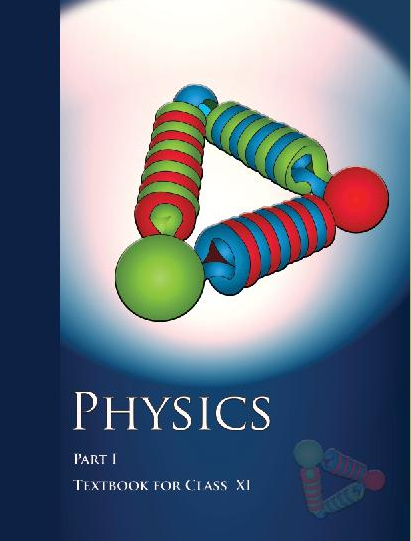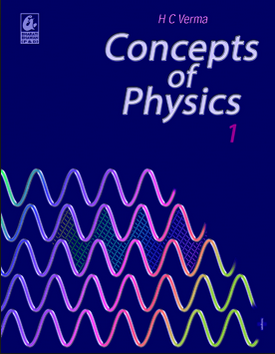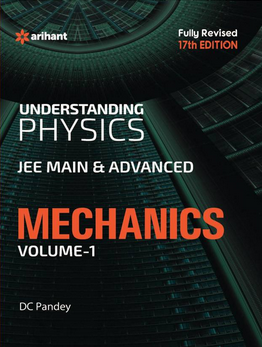# Kinematics (Weightage 3%)   Share

### Topics from Kinematics

• The frame of reference. (1 concepts)
• Motion in a straight line: Position-time graph, speed, and velocity. (18 concepts)
• Uniform and non-uniform motion, average speed and instantaneous velocity (6 concepts)
• Uniformly accelerated motion, velocity-time, position-time graphs, relations for uniformly accelerated motion. (13 concepts)
• Scalars and Vectors, Vector addition and Subtraction, Zero Vector, Scalar and Vector products, Unit Vector, Resolution of a Vector. (22 concepts)
• Relative Velocity (10 concepts)
• Motion in a plane (1 concepts)
• Projectile Motion (21 concepts)
• Uniform Circular Motion (8 concepts)

### Important Books for Kinematics

•••Exams
Articles
Questions